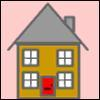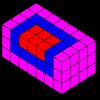Search by Topic

Resources tagged with Square numbers similar to Time of Birth:

Filter by: Content type:
Age range:
Challenge level:

There are 14 results

Broad Topics > Numbers and the Number System > Square numbersTime of Birth

Age 11 to 14 Challenge Level:

A woman was born in a year that was a square number, lived a square number of years and died in a year that was also a square number. When was she born?Triangular Triples

Age 14 to 16 Challenge Level:

Show that 8778, 10296 and 13530 are three triangular numbers and that they form a Pythagorean triple.Factors and Multiples Puzzle

Age 11 to 14 Challenge Level:

Using your knowledge of the properties of numbers, can you fill all the squares on the board?Digat

Age 11 to 14 Challenge Level:

What is the value of the digit A in the sum below: [3(230 + A)]^2 = 49280AIff

Age 14 to 18 Challenge Level:

Take a triangular number, multiply it by 8 and add 1. What is special about your answer? Can you prove it?Smith and Jones

Age 14 to 16 Challenge Level:

Mr Smith and Mr Jones are two maths teachers. By asking questions, the answers to which may be right or wrong, Mr Jones is able to find the number of the house Mr Smith lives in... Or not!Triangles Within Squares

Age 14 to 16 Challenge Level:

Can you find a rule which relates triangular numbers to square numbers?Sticky Numbers

Age 11 to 14 Challenge Level:

Can you arrange the numbers 1 to 17 in a row so that each adjacent pair adds up to a square number?Age 14 to 16 Challenge Level:

Robert noticed some interesting patterns when he highlighted square numbers in a spreadsheet. Can you prove that the patterns will continue?Generating Triples

Age 14 to 16 Challenge Level:

Sets of integers like 3, 4, 5 are called Pythagorean Triples, because they could be the lengths of the sides of a right-angled triangle. Can you find any more?Square Routes

Age 11 to 14 Challenge Level:

How many four digit square numbers are composed of even numerals? What four digit square numbers can be reversed and become the square of another number?Summing Squares

Age 14 to 16 Challenge Level:

Discover a way to sum square numbers by building cuboids from small cubes. Can you picture how the sequence will grow?Filling the Gaps

Age 14 to 16 Challenge Level:

Which numbers can we write as a sum of square numbers?Few and Far Between?

Age 14 to 18 Challenge Level:

Can you find some Pythagorean Triples where the two smaller numbers differ by 1?